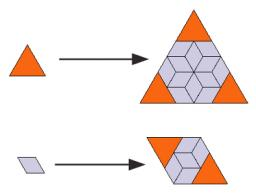# Calculate 2673

In triangle ABC, the height on the c side is 12 cm. Calculate the area of this triangle if a = 15 cm and b = 13 cm.

S =  84 cm2

### Step-by-step explanation:

Try calculation via our triangle calculator.Did you find an error or inaccuracy? Feel free to write us. Thank you!

Tips for related online calculators